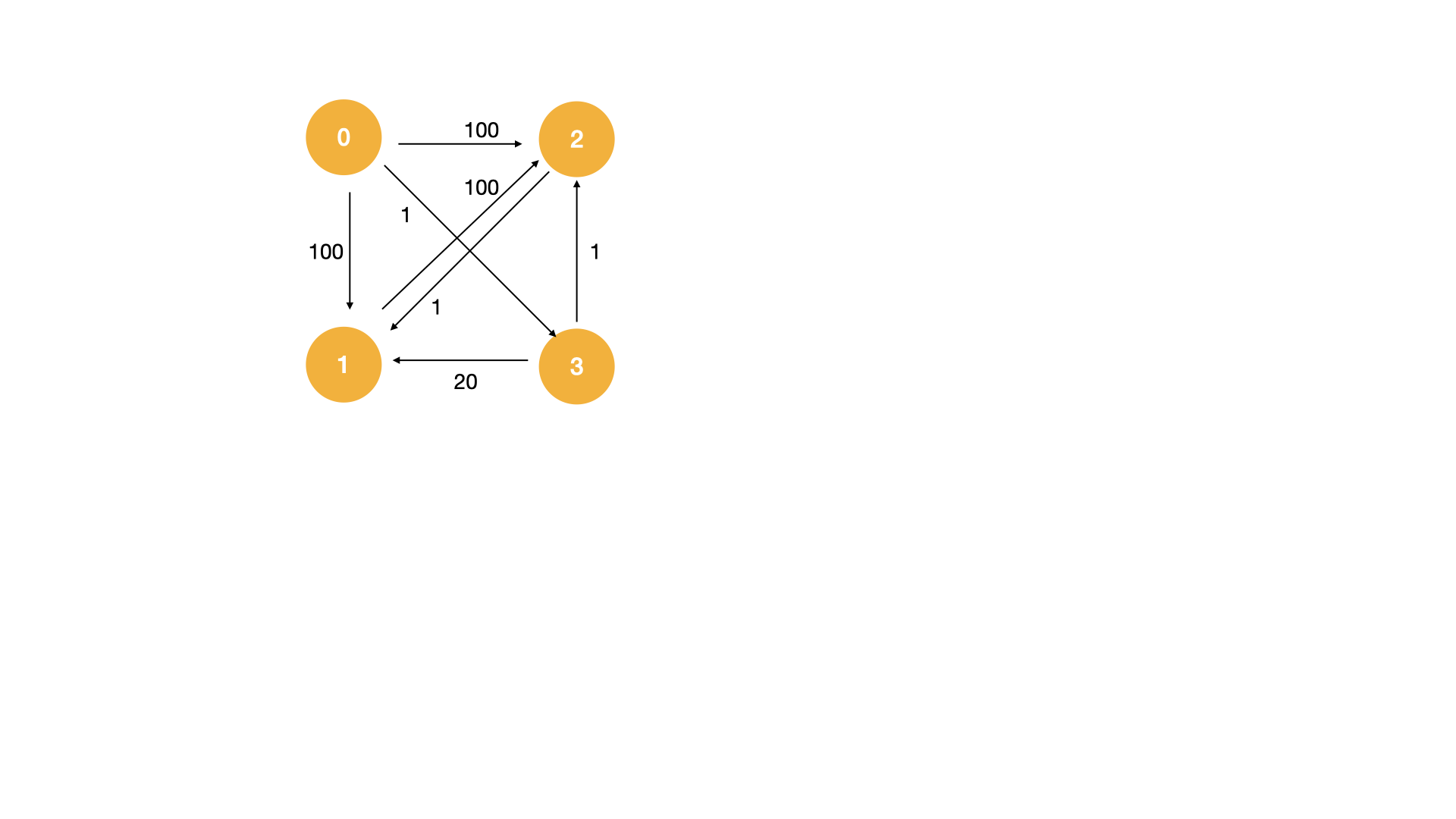# Minimum Cost to Visit Every Node in a Graph

Output the minimum cost to traverse every node in a directed weighted graph. The graph will be in the form of a 2D list where element `[i,j]` in the array denotes the weight of the directed edge from `i` to `j`. If the value is 0 then the edge doesn't exist. You do not have to end at the starting node. All edges are guaranteed to be in the range `[0,1000]`, there will not exceed 15 nodes in the graph. The starting node will always be at node 0. If a solution does not exist return -1.

### Example:

#### Input:`3`